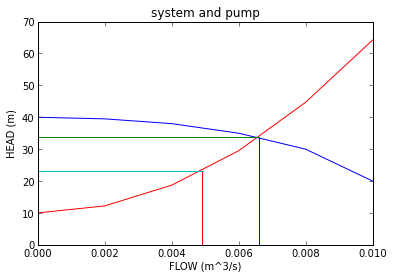# Chapter 9,Pumps¶

## Example 9.2 (part1 and part2), Page 239¶

In :
import math

#Variable Declaration
d =0.1; # m
v_r =2; # m/ s
f =0.005;
g =9.81; #m/ s ^2
pi=3.14;
L_s =2; #m
L_r =10; # m
Q1 =1.1*10**( -2) ; #m^3/ s
z_t =12; #m
z_s=5 #m
L1 =20; #m

#Calculation
Q= math.pi*d**2/4*v_r ;
H =12-70*Q-4300*Q*Q ;
k =2*g*H/ v_r/v_r-(4*f*( L_s +L_r)/d)-1;

#result
print"The head loss across the restriction orifice (in terms of velocity head)",round(k,3)

#Part2
#Finding System Head and Delivered Head
# For the case of the valve being fully open

#calculation
v_t =4*Q1/math.pi/d**2;
v_r =((2*g*( z_t-z_s )+(4*f*L1/d+1)*v_t**2) /(4*f*L_r/d+k+1))**(0.5) ;
H1 =4*f* L_r /d*v_r**2/2/ g + 4*f* L_s /d*( v_r**2+ v_t**2) /2/g+k* v_r**2/2/ g + v_r**2/2/ g;
Q= math.pi*d**2/4*( v_t+ v_r );
H2 =12 -70*Q -4300* Q**2;

#result
print" System head(m)=",round(H1,3)
print" Delivered head(m)=",round(H2,3)
print"The delivered head therefore closely matches the system head at the flow rate of 1.1*10^( -2) m^3/ s"

The head loss across the restriction orifice (in terms of velocity head) 44.863
System head(m)= 7.603
Delivered head(m)= 7.628
The delivered head therefore closely matches the system head at the flow rate of 1.1*10^( -2) m^3/ s


## Example 9.6, Page 247¶

In :
from math import pi
#Variable Declaration
NPSH =5; # m
p_v =18*10**3; # N/m^2
p_l =0.94*101.3*10**3; # N/m^2
rho =970;#kg /m^3
g =9.81; # m/ s ^2
z_s =3; # m
H_L =0.5; # m
d =3; # m
h =2.5; # m
Q =5; #m^3/h

#calculation
z1= NPSH +( p_v -p_l)/ rho /g + z_s + H_L ;
V= pi /4*d**2*(h-z1);
t=V/Q;

#result
print"Quantity of liquid delivered (m^3)=",round(V,2)
print"Time taken (h)=",round(t,2)

Quantity of liquid delivered (m^3)= 14.95
Time taken (h)= 2.99


## Example 9.8,Page 251¶

In :
#variable Declaration
N_s =0.14; #m^ ( 3 / 4 ) s ^(-3/2)
H =30; #m
p_v =7.38*10**3; #N/m^2
p_l =50*10**3; #N/m^2
rho =992; #kg /m^3
g =9.81; #m/ s ^2
H_L =0.2; #m

#calculation
NPSH =2.8* N_s **(1.33) *H;
z1= NPSH +( p_v -p_l)/ rho /g+H_L;

#result
print"The minimum level of the alarm (m)=",round(z1,3)

The minimum level of the alarm (m)= 1.967


## Example 9.10,Page 255¶

In :
%matplotlib inline
#Vabiable Declaration
import numpy as np
from pylab import *
dz =10; #z2-z1
g =9.81; #m/ s ^2
d =0.05; #m
f =0.005;
L =100; #m
N1 =1200; #rpm

# H=z2-z1+16Q^2/2/ g /%pi ^2/d ^4(4 f L/d+1)
# H=10+5.4210^5Q^2

#Calculation
Q =[0.000, 0.002, 0.004, 0.006, 0.008, 0.010];
H_p =[40.0, 39.5, 38.0, 35.0, 30.0, 20.0];
H_s =[10.0, 12.2, 18.7, 29.5, 44.7, 64.2];
figure()
plot (Q,H_p , 'b')
plot (Q,H_s , 'r')
xlabel('FLOW (m^3/s)')
ylabel('HEAD (m)')
title('system and pump')
#xtitle (" " , "Flow" , "Head")
#legend ("pump" , " system")
a =[0.0066, 0.0066];
b =[0, 33.8];

plot (a,b)
e =[0, 0.0066];
f =[33.8, 33.8];
plot (e,f)
i =[0.0049, 0.0049];
h =[0, 23];

plot (i,h)
l =[0, 0.00495];
m =[23, 23];
plot (l,m)
show()

#From graph
H1 =34.0; #m
H2 =23.0; # m
Q1 =0.0066; #m^3/ s
Q2 =0.00495; #m^3/ s
N2=N1 *( H2/H1)**(0.5) ;

#result
print "Duty point(m^3/s) =",round(Q1,4)
print "The speed of the pump to reduce the flow by 25% =",round(N2,3)

Welcome to pylab, a matplotlib-based Python environment [backend: module://IPython.zmq.pylab.backend_inline].
For more information, type 'help(pylab)'.Duty point(m^3/s) = 0.0066
The speed of the pump to reduce the flow by 25% = 986.974


## Example 9.11,Page 257¶

In :
#Varable Declaration
Q =0.05; # m^3/ s
v =2; # m/ s
f =0.005;
L_s =5; # m
d =0.178; #m
g =9.81; # m/ s ^2
L_d =20; # m
p2 =1.5*10**5; # N/m^2
p1 =0.5*10**5; # N/m^2
rho =1000; # kg /m^3
z2 =15; # m
z1 =5; # m
N1 =1500.0/60; # rps
pi=3.14;
Q =[0, 5, 10, 15, 20, 25];
H =[9.25, 8.81, 7.85, 6.48, 4.81, 2.96];
P=[- 0.96, 1.03, 1.19, 1.26, 1.45];
n1 =[0, 45, 75, 800, 75, 50];
H =27.96; #m
H1 =6.48; #m
Q1 =0.015; #m^3/ s
Q =0.05; #m^3/ s
D1 =0.15; #m
n =0.80;

#calculation
d =(4* Q/ pi /v)**(0.5) ;
H_f_s =4* f* L_s /d*v **2/2/ g;
H_f_d =4* f* L_d /d*v **2/2/ g;
H =1/(1 -0.25) *(( p2 -p1)/ rho /g + v **2/2/ g + z2 - z1 +H_f_s + H_f_d );
# n=rho gQH/P
N=N1 *(H/H1) **(0.75) *( Q1/Q) **(0.5) ;
D=D1 *(Q*N1/Q1/N)**(0.2) ;
P= rho *g*Q*H/n;

#result
print " Differential Head (m) =",round(H,3);
print "impeller diameter (m)=",round(D,3)
print "The rotational speed at maximum efficiency (rps)=",round(N,3)
print "Power input to the pump (W)=",round(P,3)

 Differential Head (m) = 27.958
impeller diameter (m)= 0.173
The rotational speed at maximum efficiency (rps)= 40.992
Power input to the pump (W)= 17141.964


## Example 9.12,Page 259¶

In :
#Variable Declaration
N =2000.0/60; #rps
Q =50.0/3600; #m^3/ s
g =9.81; #m/ s ^2
H =5.0; #m

#Calculation
S_n =N*Q **(0.5) /(g)**(0.75)/(H) **(0.75) ;

#result
print " Suction specific speed =",round(S_n,3)

 Suction specific speed = 0.212


## Example 9.14,Page 264¶

In :
# Variable Declaration
A =0.01; #m^2
L =0.3; # m
N =60/60; # rps
V_act =10.6/3600; # m^3/ s
rho =1000; # kg /m^3
g =9.81; # m/ s ^2
Q =10.6/3600; # m^3/ s
H =15; # m

#calculation
V=A*L*N;
Cd= V_act /V;
P= rho *g*Q*H;

#result
print " Coeff. of Discharge =",round(Cd,2)
print "The power required (W)=",round(P,3)

 Coeff. of Discharge = 0.98
The power required (W)= 433.275

In [ ]: